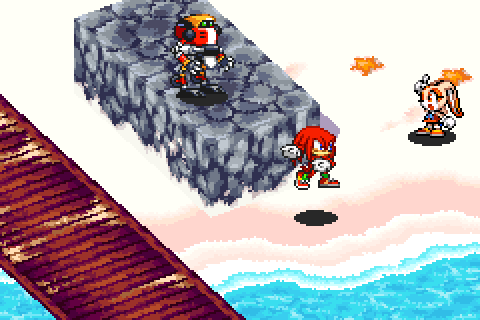Sonic Battle (GBA) Renderer Series - Camera

Posted on November 14, 2018

Welcome to the sixth article of the Sonic Battle (GBA) Renderer series.Camera

Background info

A general camera system is described in Joey de Vries’ learnopengl.com series:

Specific

For reference, here’s the coordinate system used in this series.

Our camera has very little “primary” state. The state that the user can set is:

• Position in 3D space
• Heading (rotation around the Z axis)
• Pitch (rotation around the X axis)

It also contains “secondary” state which is derived once per frame from the primary state:

• Facing direction in 3D
• Facing direction in 2D (on the XY plane)
• Transformation matrix and its inverse (the inverse is the conventional view matrix)

Transformation matrix

• Create translation matrix using position
• Apply heading rotation
• Apply pitch rotation

In conventional matrix notation this would be: translationMatrix * headingMatrix * pitchMatrix.

The GLM math library takes care of the specifics. A few details:

• Base axis rotation (like rotation around the Z or X axis) is a special case which can be computed cheaply. GLM’s euler angle functions are used for that.
• The inverse can be computed from a mat3, then casted back to mat4 and multiplied with the opposite translation matrix (mat3 inverse is much, much faster than mat4 inverse)

Final result:

mat4 camPitch = eulerAngleX(Pitch)
mat4 camOrientation = camHeading * camPitch
Transform = translate(camOrientation, Pos)

mat4 camOrientationInverse = mat4(inverse(mat3(camOrientation)))
TransformInverse = translate(camOrientationInverse, -Pos)

eulerAngleZ and eulerAngleX both do a cos and a sin call to construct their base axis rotation matrix.

Facing direction in 3D

• The default direction is vec(0, 1, 0, 0)
• The 3D direction is transformMatrix * defaultDirection

Which simplifies to become the second column of the transformation matrix.

Facing direction in 2D

(Using the trigonometric circle.)

Alternatively we could normalize a vector made from the 3D direction’s x and y components.

Fixed-point math

Once the camera data is computed trigonometric functions aren’t used at all during the rest of the rendering.

Only multiplication and addition (these two operations also cover matrix multiplication) are used from this point forward.

This makes software-based fixed-point math accurate and fast (because any non-simple math operations require quicker approximations which compromise accuracy and are still pretty slow in the end).

Note - canvas space

I’ll ommit the subject of canvas/screen space (see this note for details). For the sake of simplicity the series will treat view/camera space as the final space for rendering.

The next article deals with walls and tilemaps.